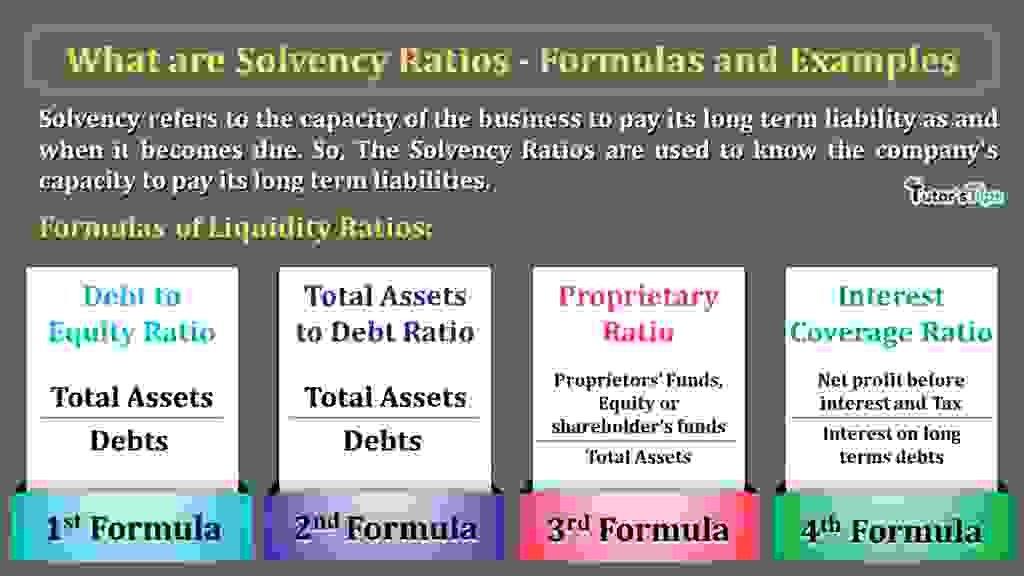# What are Solvency Ratios – Formulas and ExamplesSolvency Ratios show the capacity of the company to repay its long term liabilities by realising the total assets. It is also the basic ratio of the accounting ratios to know the companies financial position.

## Meaning of Solvency Ratios:

Solvency refers to the capacity of the business to pay its long term liability as and when it becomes due. So, The Solvency Ratios are used to know the company’s capacity to pay its long term liabilities.

To pay the long term liabilities means paid the total due amount of long term liabilities by realising amount from the company’s total assets.

## Why do we need to Calculate Solvency Ratios?

Solvency ratio provides us with information about the financial stability of the business. The high Solvency ratio ensures that the company is in a good position to meet its long term financial obligation and the low Solvency  ratio shows the low capacity of the firm to meet its long term financial obligation and it will lead to bankruptcy and fall down the credit rating of the business.

## The formula of Solvency Ratios:

To Check out the liquidity of the business we can use the following three formulas:

### 1. Debt to Equity Ratio:

The Debt to Equity ratio is used to compare the Equity (i.e. shareholder’s funds) with debts (i.e. outsider’s liabilities) of the business.

###### Formula to Calculate Debt to Equity Ratio:
 Debt to Equity Ratio = Debts Equity

### 2. Total Assets to Debt Ratio

The Total Assets to Debt ratio is used to compare the Total assets with debts (i.e. outsider’s liabilities) of the business.

###### Formula to Calculate Total Assets to Debt Ratio:
 Total Assets to Debt Ratio = Total Assets Debts

### 3. Proprietary Ratio:

The Proprietary ratio is used to compare the Proprietors’ Funds, Equity or shareholder’s funds with Total assets of the business.

###### Formula to calculate Absolute liquid or cash Ratio:
 Proprietary Ratio = Proprietors’ Funds, Equity or shareholder’s funds Total Assets

### 4. Interest Coverage Ratio:

The Interest Coverage ratio is used to compare the Net profit before interest and Tax with interest on long terms debts of the business.

###### Formula to calculate Absolute liquid or cash Ratio:
 Interest Coverage Ratio = Net profit before interest and Tax interest on long terms debts

We will get ratio in times.

Thanks for reading the topic.

please comment your feedback whatever you want. If you have any questions, please ask us by commenting.

References: –